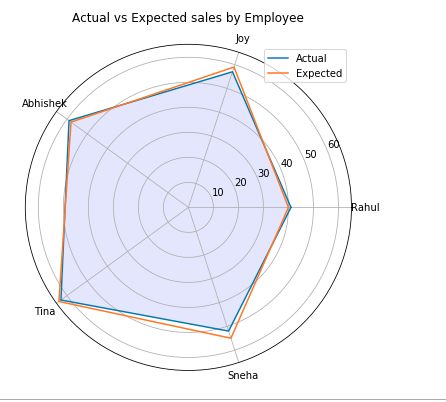Matplotlib.pyplot.thetagrids() in Python

• Last Updated : 29 Jul, 2021

Matplotlib is a plotting library of Python programming language and its numerical mathematics module is NumPy. matplotlib.pyplot is a collection of command style functions that make matplotlib work like the MATLAB Tool. Each of the pyplot functions makes certain changes to a figure: e.g., creating a figure, creating a plot area in a figure, plots some lines in a plotting area or decorate the plot with labels, etc.

Matplotlib.pyplot.thetagrids()

Set the theta locations of the gridlines in the polar plot. If no arguments are passed, it returns a tuple (lines, labels) where lines are an array of radial gridlines (Line2D instances) and labels is an array of tick labels (Text instances):

Syntax: lines, labels = thetagrids(angles, labels=None, fmt=’%d’, frac = 1.5)
Parameters:

• Angles:

set the angles to the place of theta grids (these gridlines are equal along the theta dimension)

• labels:

if not None, then it is len(angles) or list of strings of the labels to use at each angle. If labels are None, the labels will be fmt%angle.

• frac: It is the fraction of the polar axes radius at the place of label (1 is the edge). e.g., 1.25 is outside the axes and 0.75 is inside the axes.

Return Type: Return value is a list of tuples (lines, labels)
Note: lines are Line2D instances, labels are Text instances.

• Example:

Python3

 import matplotlib.pyplot as pltimport numpy as np    employee = ["Rahul", "Joy", "Abhishek",            "Tina", "Sneha"]  actual = [41, 57, 59, 63, 52, 41]expected = [40, 59, 58, 64, 55, 40]    # Initialing the spiderplot by # setting figure size and polar# projectionplt.figure(figsize =(10, 6))plt.subplot(polar = True)    theta = np.linspace(0, 2 * np.pi, len(actual))    # Arranging the grid into number # of sales into equal parts in# degreeslines, labels = plt.thetagrids(range(0, 360, int(360/len(employee))),                                                         (employee))    # Plot actual sales graphplt.plot(theta, actual)plt.fill(theta, actual, 'b', alpha = 0.1)    # Plot expected sales graphplt.plot(theta, expected)    # Add legend and title for the plotplt.legend(labels =('Actual', 'Expected'),           loc = 1)plt.title("Actual vs Expected sales by Employee")    # Display the plot on the screenplt.show()

Output:My Personal Notes arrow_drop_up